New update is available. Click here to update.
Topics

# Cycle Detection in a Singly Linked List

Moderate0/80
Average time to solve is 15m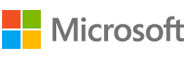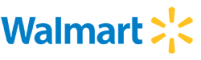+113 more companies

## Problem statement

You are given a Singly Linked List of integers. Return true if it has a cycle, else return false.

A cycle occurs when a node's next points back to a previous node in the list.

Example:
``````In the given linked list, there is a cycle, hence we return true.
``````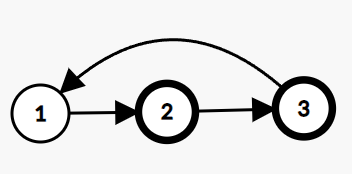Detailed explanation ( Input/output format, Notes, Images )
Sample Input 1 :
``````1 2 3 4 -1
1
``````

Sample Output 1 :
``````true
``````

Explanation of Sample Input 1:
``````The linked list given in the input is as follows:
``````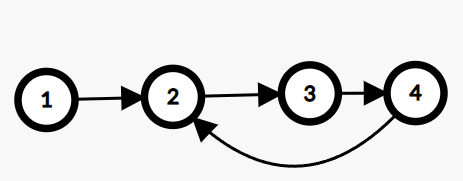Sample Input 2 :
``````1 2 3 4 -1
0
``````

Sample Output 2 :
``````true
``````

Explanation of Sample Input 2:
``````The linked list given in the input is as follows:
``````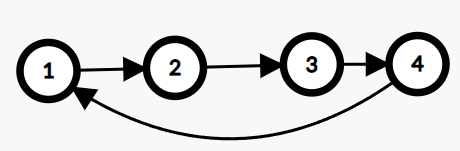Sample Input 3 :
``````5 -1
-1
``````

Sample Output 3 :
``````false
``````

Explanation of Sample Input 3:
`````` The linked list given in the input is as follows:
``````Expected Time Complexity:
``````Try to solve this problem in O(n).
``````

Expected Space Complexity:
``````Try to solve this problem in O(1).
``````

Constraints :
``````0 <= n <= 10^6
-1 <= pos < n
-10^9 <= data <= 10^9 and data != -1

Where 'n' is the size of the singly linked list, 'pos' represents the position (0-indexed) in the linked list where the tail connects to, and 'data' is the Integer data of the singly linked list.

Time Limit: 1 sec
``````Console